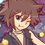# Combinatorics (Thailand Math POSN 2nd round)

Write a full solution.

1.) In a party of 100 people, each people are known by even number of people. Prove that there exists a set of 3 people that are known by the same number of people.

2.1) An ordinary deck of cards consists of 52 cards - 4 suits, 13 cards each suits. Take out all cards with $J,Q,K,A$. Find the number of different ways to choose 4 cards from the leftover such that the sum of all 4 numbers on chosen cards is 30, and none of the cards chosen have the same suit.

2.2) Find the number of different base-4 $n$-digit numbers such that

• there exists the digit 0 on any place (0 can be at the first digit)
• there are even number of digit 0
• there are no more than 1 number of digit 1

3.) Let $k,n$ be a natural number. Let $A = \{1,2,\dots,kn\}$. Find the least number to choose the elements from $A$ such that we guarantee that there exists $k$ consecutive numbers within the chosen number.

4.) Write down each digits from 1 to 9 in the $14 \times 14$ table, such that the adjacent cells (horizontal, vertical, diagonal) must be coprime to each other. Prove that there exists 1 digit that appears on the table at least 33 times.

5.) Let $S$ be a set of 8-digit number of all possible permutations of 1,2,3,4,6,7,8,9. Let the number from set $S$ nicely arranged if there exists a pair adjacent digits that has an absolute difference of 5. Compare the number of nicely arranged numbers and not nicely arranged.

(If possible, find the number of both nicely arranged and not nicely arranged.)

Example: 13726489 is nicely arranged because the adjacent number 72 have a difference of 5, but 13468792 is not nicely arranged.

This note is a part of Thailand Math POSN 2nd round 2015Note by Samuraiwarm Tsunayoshi
5 years, 7 months ago

This discussion board is a place to discuss our Daily Challenges and the math and science related to those challenges. Explanations are more than just a solution — they should explain the steps and thinking strategies that you used to obtain the solution. Comments should further the discussion of math and science.

When posting on Brilliant:

• Use the emojis to react to an explanation, whether you're congratulating a job well done , or just really confused .
• Ask specific questions about the challenge or the steps in somebody's explanation. Well-posed questions can add a lot to the discussion, but posting "I don't understand!" doesn't help anyone.
• Try to contribute something new to the discussion, whether it is an extension, generalization or other idea related to the challenge.

MarkdownAppears as
*italics* or _italics_ italics
**bold** or __bold__ bold
- bulleted- list
• bulleted
• list
1. numbered2. list
1. numbered
2. list
Note: you must add a full line of space before and after lists for them to show up correctly
paragraph 1paragraph 2

paragraph 1

paragraph 2

[example link](https://brilliant.org)example link
> This is a quote
This is a quote
    # I indented these lines
# 4 spaces, and now they show
# up as a code block.

print "hello world"
# I indented these lines
# 4 spaces, and now they show
# up as a code block.

print "hello world"
MathAppears as
Remember to wrap math in $$ ... $$ or $ ... $ to ensure proper formatting.
2 \times 3 $2 \times 3$
2^{34} $2^{34}$
a_{i-1} $a_{i-1}$
\frac{2}{3} $\frac{2}{3}$
\sqrt{2} $\sqrt{2}$
\sum_{i=1}^3 $\sum_{i=1}^3$
\sin \theta $\sin \theta$
\boxed{123} $\boxed{123}$

Sort by:

I think 1) is wrong. Place the 100 people in a line and label them 1,2,3... Group them like this, [1,2],[3,4],[5,6]... Now if every group knows the everyone from the groups after them, for example, [51,52] know [53,54],[55,56]... Then all people are known by an even number of people and no three people are known by the same number of people.

- 5 years, 7 months ago

The original question has a lot of ambiguity though. I think it has to be mutual relationship that $A$ knows $B$ then $B$ also has to know $A$. I'm changing what I stated so that the question will be right.

- 5 years, 7 months ago